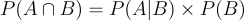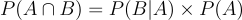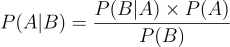16 Oct 2023

Posted on:

10 Oct 2023

0

# Resolved:I don't get the rationale of some concepts and need some clarification

In the 2nd lecture of the course it's said that the probability of two independent events occurring at the same time is the product of the individual probabilities which is been clarified here that this is the probability of their intersection.

My first question is if two events are independent how can they happen at the same time? being independent means there is no intersection so the probability of both happening at the same time should always equal 0? and the formula of the product of the individual probabilities should be called the probability of dependent event?

Also if p(A|B) = p(A^B)/p(B) and P(A^B) = p(A) * p(B)
So, p(A|B) = p(A) * p(B) / p(B)  wouldn't p(B) in the denominator cross out the one in the numerator so p(A|B) always equal p(A)?

Instructor
Posted on:

11 Oct 2023

0

Hey Sayed,

Thanks for reaching out.

Regarding your first question, an example of two independent events that can happen at the same are drawing a Diamond and drawing an Ace from a deck of cards. For a detailed explanation on why that is true, please refer to the following thread:
https://365datascience.com/q/782afba348

Concerning your second question, let's recall that P(A|B) reads "the probability of event A happening given that event B has happened". Therefore, if events A and B are independent, event A would not be affected by event B and your derivation would be completely correct. Very rightfully, you obtain that P(A|B) = P(A). Similarly, if the same condition holds (events A and B are independent), then P(B|A) = P(B) since event A would not affect the outcome of event B.

Note that if events A and B are dependent, then your derivation wouldn't hold since the expression

P(A ∩ B) = P(A) × P(B)

is no longer valid. It is only true for independent events.

Hope this helps!

Kind regards,

365 Hristina

Posted on:

14 Oct 2023

0

Hi,

I searched about P(A ∩ B) for dependent events and found that for dependent events:

P(A ∩ B) = P(A) * P(B after A) and that P(B after A) is the same as P(B|A) !!

So, the formula of P(B|A) = P(A) * P(B|A) / P(A) ??!

Does that mean dealing with conditional probabiliy is more of playing with Venn diagrams and sample spaces than a mathematical formula we can plug numbers in?

Instructor
Posted on:

16 Oct 2023

0

Hey again Sayed,

Note the following:

P(A ∩ B) represents the probability of both events A and B occurring simultaneously;

P(A|B) is the probability of event A happening given that event B has already happened. The symbol | is read as "given".

It is true thatandEquating the right-hand sides of both equations yields Bayes' theorem (discussed in Section 3: Bayesian Inference)I'm uncertain how to address your final question, but I hope that the formulas provided above will clarify any confusion. :)

Kind regards,

365 Hristina